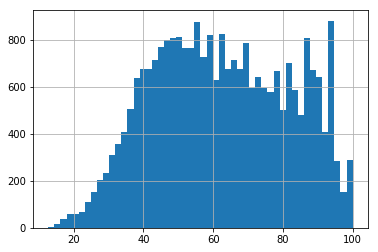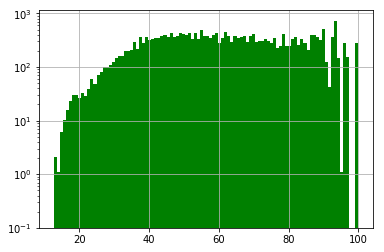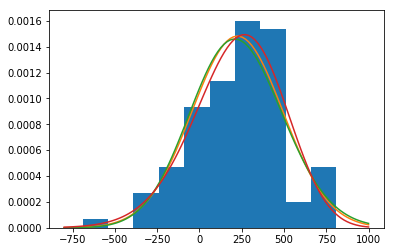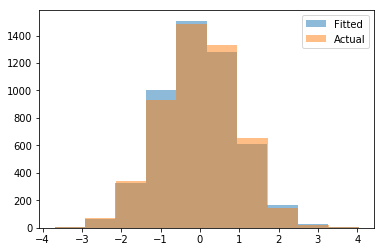import pandas as pd
import matplotlib.pyplot as plt
import datetime
import numpy as np
%matplotlib inline
from scipy import stats
from pylab import plot,show,hist,figure,title
from scipy.stats import norm


dataw = pd.read_csv('weather.csv') # source: https://raw.githubusercontent.com/hadley/nycflights13/master/data-raw/weather.csv

dataw.head()

origin year month day hour temp dewp humid wind_dir wind_speed wind_gust precip pressure visib time_hour
0 EWR 2013 1 1 1 39.02 26.06 59.37 270.0 10.35702 NaN 0.0 1012.0 10.0 2013-01-01T06:00:00Z
1 EWR 2013 1 1 2 39.02 26.96 61.63 250.0 8.05546 NaN 0.0 1012.3 10.0 2013-01-01T07:00:00Z
2 EWR 2013 1 1 3 39.02 28.04 64.43 240.0 11.50780 NaN 0.0 1012.5 10.0 2013-01-01T08:00:00Z
3 EWR 2013 1 1 4 39.92 28.04 62.21 250.0 12.65858 NaN 0.0 1012.2 10.0 2013-01-01T09:00:00Z
4 EWR 2013 1 1 5 39.02 28.04 64.43 260.0 12.65858 NaN 0.0 1011.9 10.0 2013-01-01T10:00:00Z
dataw.humid.hist(bins=50) # bins changes the y-axis(?)

<matplotlib.axes._subplots.AxesSubplot at 0x10bc5b9e8># To add the log scale in the y-axis
fig, ax = plt.subplots()
dataw.humid.hist(ax=ax, bins=100,color='green',bottom=0.1)  # bins do not get updated when I set the value to e.g. 50?
ax.set_yscale('log')## Fitting a distribution¶

# See also: http://danielhnyk.cz/fitting-distribution-histogram-using-python/

# create some normal random noisy data
ser = 50*np.random.rand() * np.random.normal(10, 10, 100) + 20

# plot normed histogram
plt.hist(ser, normed=True)

# find minimum and maximum of xticks, so we know
# where we should compute theoretical distribution
xt = plt.xticks()
xmin, xmax = min(xt), max(xt)
lnspc = np.linspace(xmin, xmax, len(ser))

# lets try the normal distribution first
m, s = stats.norm.fit(ser) # get mean and standard deviation
pdf_g = stats.norm.pdf(lnspc, m, s) # now get theoretical values in our interval
plt.plot(lnspc, pdf_g, label="Norm") # plot it

# exactly same as above
ag,bg,cg = stats.gamma.fit(ser)
pdf_gamma = stats.gamma.pdf(lnspc, ag, bg,cg)
plt.plot(lnspc, pdf_gamma, label="Gamma")

# guess what :)
ab,bb,cb,db = stats.beta.fit(ser)
pdf_beta = stats.beta.pdf(lnspc, ab, bb,cb, db)
plt.plot(lnspc, pdf_beta, label="Beta")

plt.show()

/Users/sarasua/anaconda3/lib/python3.6/site-packages/scipy/stats/_continuous_distns.py:412: RuntimeWarning: invalid value encountered in sqrt
sk = 2*(b-a)*np.sqrt(a + b + 1) / (a + b + 2) / np.sqrt(a*b)
/Users/sarasua/anaconda3/lib/python3.6/site-packages/scipy/optimize/minpack.py:161: RuntimeWarning: The iteration is not making good progress, as measured by the
improvement from the last ten iterations.
warnings.warn(msg, RuntimeWarning)# See also:

class Distribution(object):

def __init__(self,dist_names_list = []):
self.dist_names = ['norm','lognorm','expon','pareto']
self.dist_results = []
self.params = {}

self.DistributionName = ""
self.PValue = 0
self.Param = None

self.isFitted = False

def Fit(self, y):
self.dist_results = []
self.params = {}
for dist_name in self.dist_names:
dist = getattr(stats, dist_name)
param = dist.fit(y)

self.params[dist_name] = param
#Applying the Kolmogorov-Smirnov test
D, p = stats.kstest(y, dist_name, args=param);
self.dist_results.append((dist_name,p))

#select the best fitted distribution
sel_dist,p = (max(self.dist_results,key=lambda item:item))
#store the name of the best fit and its p value
self.DistributionName = sel_dist
self.PValue = p

self.isFitted = True
return self.DistributionName,self.PValue

def Random(self, n = 1):
if self.isFitted:
dist_name = self.DistributionName
param = self.params[dist_name]
#initiate the scipy distribution
dist = getattr(stats, dist_name)
return dist.rvs(*param[:-2], loc=param[-2], scale=param[-1], size=n)
else:
raise ValueError('Must first run the Fit method.')

def Plot(self,y):
x = self.Random(n=len(y))
plt.hist(x, alpha=0.5, label='Fitted')
plt.hist(y, alpha=0.5, label='Actual')
plt.legend(loc='upper right')

r = norm.rvs(size=5000)

dst = Distribution()
dst.Fit(r)
dst.Plot(r)

/Users/sarasua/anaconda3/lib/python3.6/site-packages/scipy/stats/_distn_infrastructure.py:2303: RuntimeWarning: invalid value encountered in double_scalars
Lhat = muhat - Shat*mu# Normality tests: https://machinelearningmastery.com/a-gentle-introduction-to-normality-tests-in-python/

# generate gaussian data
from numpy.random import seed
from numpy.random import randn
from numpy import mean
from numpy import std
# seed the random number generator
seed(1)
# generate univariate observations
data = 5 * randn(100) + 50
# summarize
print('mean=%.3f stdv=%.3f' % (mean(data), std(data)))

mean=50.303 stdv=4.426

# Shapiro-Wilk Test - for smaller data sets around thousands of records
from numpy.random import seed
from numpy.random import randn
from scipy.stats import shapiro
# seed the random number generator
seed(1)
# generate univariate observations
data = 5 * randn(100) + 50
# normality test
stat, p = shapiro(data)
print('Statistics=%.3f, p=%.3f' % (stat, p))
# interpret
alpha = 0.05
if p > alpha:
print('Sample looks Gaussian (fail to reject H0)')
else:
print('Sample does not look Gaussian (reject H0)')

Statistics=0.992, p=0.822
Sample looks Gaussian (fail to reject H0)

# normaltest() function in SciPy - So easy!

# D'Agostino and Pearson's Test
from numpy.random import seed
from numpy.random import randn
from scipy.stats import normaltest
# seed the random number generator
seed(1)
# generate univariate observations
data = 5 * randn(100) + 50
# normality test
stat, p = normaltest(data) #dataw.humid
print('Statistics=%.3f, p=%.3f' % (stat, p))
# interpret
alpha = 0.05
if p > alpha:
print('Sample looks Gaussian (fail to reject H0)')
else:
print('Sample does not look Gaussian (reject H0)')

Statistics=nan, p=nan
Sample does not look Gaussian (reject H0)

import pandas as pd

# Anderson-Darling Test
from numpy.random import seed
from numpy.random import randn
from scipy.stats import anderson
# seed the random number generator
seed(1)
# generate univariate observations
data = 5 * randn(100) + 50

# normality test
print(pd.Series(data).index)
result = anderson(pd.Series(data)) #dataw.humid
print('Statistic: %.3f' % result.statistic)
p = 0
for i in range(len(result.critical_values)):
sl, cv = result.significance_level[i], result.critical_values[i]
if result.statistic < result.critical_values[i]:
print('%.3f: %.3f, data looks normal (fail to reject H0)' % (sl, cv))
else:
print('%.3f: %.3f, data does not look normal (reject H0)' % (sl, cv))

0    58.121727
1    46.941218
2    47.359141
3    44.635157
4    54.327038
dtype: float64
RangeIndex(start=0, stop=100, step=1)
Statistic: 0.220
15.000: 0.555, data looks normal (fail to reject H0)
10.000: 0.632, data looks normal (fail to reject H0)
5.000: 0.759, data looks normal (fail to reject H0)
2.500: 0.885, data looks normal (fail to reject H0)
1.000: 1.053, data looks normal (fail to reject H0)


What the author of the post recommends (copied and pasted from https://machinelearningmastery.com/a-gentle-introduction-to-normality-tests-in-python/):

What Test Should You Use? We have covered a few normality tests, but this is not all of the tests that exist.

So which test do you use?

I recommend using them all on your data, where appropriate.

The question then becomes, how do you interpret the results? What if the tests disagree, which they often will?

Hard Fail Your data may not be normal for lots of different reasons. Each test looks at the question of whether a sample was drawn from a Gaussian distribution from a slightly different perspective.

A failure of one normality test means that your data is not normal. As simple as that.

You can either investigate why your data is not normal and perhaps use data preparation techniques to make the data more normal.

Or you can start looking into the use of nonparametric statistical methods instead of the parametric methods.

Soft Fail If some of the methods suggest that the sample is Gaussian and some not, then perhaps take this as an indication that your data is Gaussian-like.

In many situations, you can treat your data as though it is Gaussian and proceed with your chosen parametric statistical methods.

## Proportions difference test¶

x = pd.Series([6,3,4,6])

d1 = pd.Series(([80,100,20,10]))
d2= pd.Series([60,80,60,10])
data = pd.DataFrame()
data['exp1a'] = d1
data['exp1b'] = d2
data.index = ['a','b','c','d']

exp1a exp1b
a 80 60
b 100 80
c 20 60
d 10 10









## AVG difference test¶